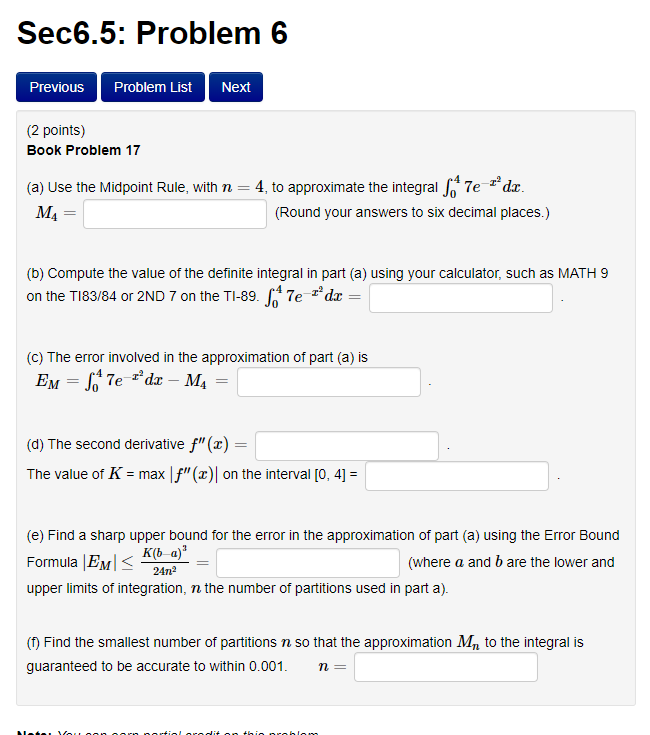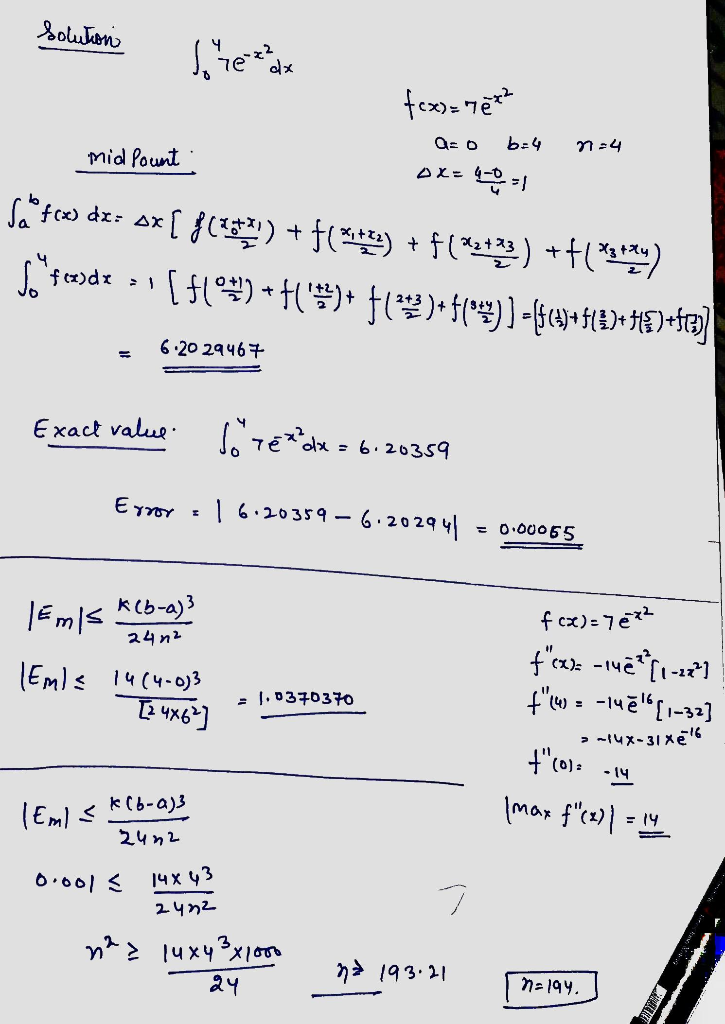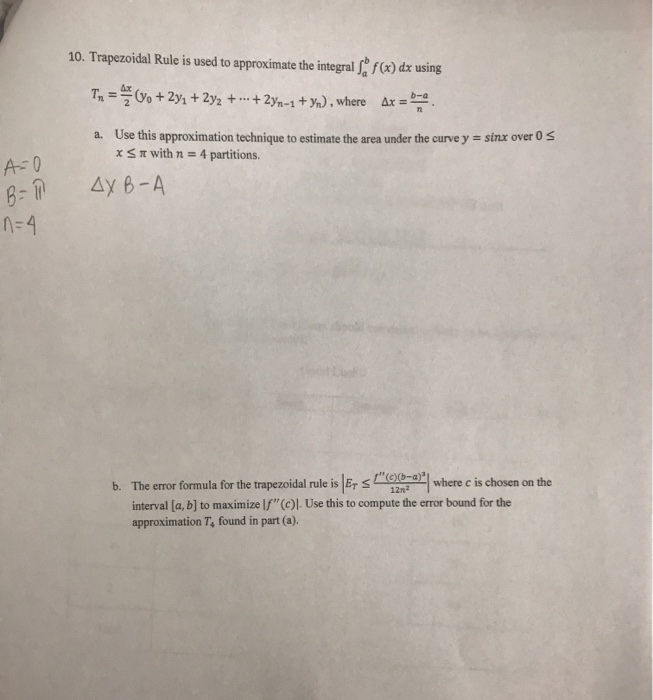# Sec6.5: Problem 6 Previous Problem List Next (2 points) Book Problem 17 4, to approximate the integral 7e dx (a) Use th...Sec6.5: Problem 6 Previous Problem List Next (2 points) Book Problem 17 4, to approximate the integral 7e dx (a) Use the Midpoint Rule, with n MA (Round your answers to six decimal places.) (b) Compute the value of the definite integral in part (a) using your calculator, such as MATH 9 on the TI83/84 or 2ND 7 on the TI-89. 7edx (c) The error involved in the approximation of part (a) is Ем — Те ах Ма (d) The second derivative f"(x) The value of K max | f" (x)| on the interval [0, 4] = (e) Find a sharp upper bound for the error in the approximation of part (a) using the Error Bound къ а)" Formula |EM upper limits of integration, n the number of partitions used in part a) (where a and b are the lower and 24n2 () Find the smallest number of partitions n so that the approximation M, to the integral is guaranteed to be accurate to within 0.001 n Aoe Vou oon ortiol rodito taio oroblon##### Add Answer of: Sec6.5: Problem 6 Previous Problem List Next (2 points) Book Problem 17 4, to approximate the integral 7e dx (a) Use th...10. Trapezoidal Rule is used to approximate the integral f(a) dx using 1- (yo +2y1 + 2y2 + x-na b-a + 2yn-1 +%),where Use this approximation technique to estimate the area under the curve y = sinx over。 a. π with n 4 partitions. x A 0 B: @ Δy B-A b. The error formula for the trapezoidal rule is RSL (12ba)1 where cischosen on the interval [a, b] to maximize lf" (c)l. Use this to compute the error bound...